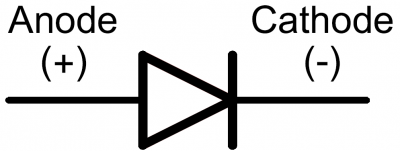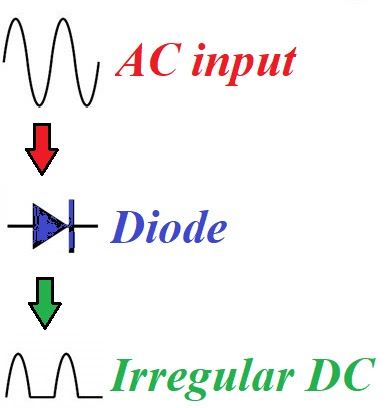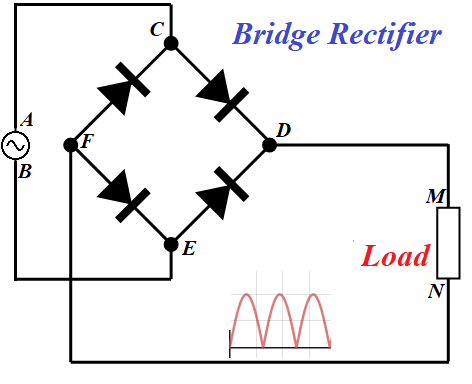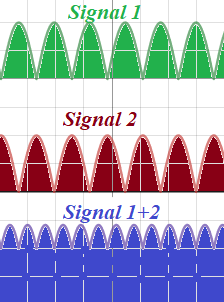## Monday, February 22, 2021

Notes to a video lecture on http://www.unizor.com

Relativity - Transformation of Space-Time Coordinates
(notes to item #3 of Einstein's "Electrodynamics")

The following is an example of how a system of linear equations can be used to derive formulas of special theory of relativity. Albert Einstein has derived these formulas in his "Electrodynamics" in a more physical, more intuitive way. The following is pure mathematics and, as such, causes much less problems in understanding.

Assumptions:
1. Assume we have two systems of coordinates, one stationary with coordinates {X,T} (assuming for simplicity all the movements will occur in one space dimension along X-axis and one time dimension) and another with coordinates {x,t} moving along the X-axis with constant speed V.
2. Assume that at time T=0 systems coincide (i.e. X=0, t=0 and x=0).
3. Assume that the speed of something, as measured in both stationary and moving systems is the same and equal to C regardless of the direction of the movement (that "something" is the light in vacuum, but it's physical characteristics are unimportant)
4. Assume further that we are looking for linear orthogonal (i.e. preserving the distance between points and angles between vectors) transformation of coordinates from (X,T) to (x,t) that satisfies the above criteria. What would this transformation be?

Linear transformation from {X,T} system to {x,t} system should look like this:
x = pX + qT
t = rX + sT
where p, q, r and s are 4 unknown coefficients of transformation, which we are going to determine by constructing a system of 4 linear equations with them.

We should not add any constants into above transformations since {X=0,T=0} should transform into {x=0,t=0}.

A. Notice that the property of orthogonality is needed to preserve geometry (i.e. no deformation) and, therefore, to preserve the form of all physical equations of motion. As it is well known, orthogonal transformations have determinant of the matrix of coefficients equal to 1, i.e.
ps − qr = 1. An unfamiliar with this property student can study this subject separately (we at Unizor plan to include this into corresponding topic on vectors).
The above is the first equation to determine unknown coefficients. It's not linear, it is rather quadratic, but the rest of the equations will be linear and that's why we included this particular problem in the topic dedicated to linear systems.
We need three more equations to determine all the unknown coefficients.

B. Since moving system moves along X-axis with speed V, its beginning of coordinate (point x=0) must at the moment of time T be on a distance VT from the beginning of coordinates of a stationary system. Hence, if X=VT, x=0 for any T. From this and the first transformation equation x = pX + qT we derive:
0 = pVT + qT or
0 = (pV + q)T.

Since this equality is true for any T,
pV + q = 0
and, unconditionally, q = −pV.
This is the second equation for our unknown coefficients.

C. Since the speed of light C is the same in both systems {X,T} and {x,t}, an equation of its motion in the stationary system must be X = CT and in the moving system x = Ct. Therefore, if X=CT, then x=Ct. Put X=CT into equations of transformation of coordinates. We get x = pCT + qT, t = rCT + sT. Substitute these expressions into x=Ct:
pCT + qT = rC2T + sCT.
Reduce by T,
pC + q = rC2 + sC.
This is the third equation for unknown coefficients.

D. Repeat the logic of a previous paragraph for the light moving in the opposite direction with a speed −C. We get, if X = −CT, then x = −Ct. Therefore, x = −pCT + qT, t = −rCT + sT and (since x = −Ct)
−pCT + qT = rC2T − sCT.
Reduce by T,
−pC + q = rC2 − sC.
This the fourth equation for unknown coefficients.

So, this is the system of equations for 4 unknown coefficients of transformation p, q, r, s:
(a) ps − qr = 1
(b) q = −pV
(c) pC + q = rC2 + sC
(d) −pC + q = rC2 − sC

It's not exactly linear, but it has sufficient number of linear equations (all but one) to solve it using the known methodology. Let's solve this system of equations by combining the methods of substitution and elimination. We will express unknown variables q, r, s in terms of p using equations (b), (c) and (d). Then we will substitute them into (a) to get an equation for p. Solving it will allow to evaluate all other unknowns.

E. From (c) and (d), adding and subtracting these equations, we get:
2q = 2rC2, therefore q = rC2
2pC = 2sC, therefore p = s

Since from (b) q = −pV,
−pV = rC2 and r = −pV/C2.

Now all coefficients of a transformation are expressed in terms of one unknown coefficient p. To get the value of p, use the first equation (a).

F. Substituting q, r and s, expressed in terms of p, into an equation (a) ps − qr = 1, we get:
p2 − (−pV)·(−pV)/C2 = 1, therefore
p2·(1 − V2/C2) = 1 and
p = 1/√1−V2/C2 .

From this all other coefficients of a transformation matrix are derived:
q = −V/√1−V2/C2
r = −(V/C2)/√
1−V2/C2
s = 1/√
1−V2/C2

G. The final transformation matrix looks exactly like in Einstein's article on electrodynamics, but seems to be much simpler to arrive at and the derivation is strictly mathematical.
x = (1/√1−V2/C2 )·X − (V/√1−V2/C2 )·T
t = ((−V/C2)/√
1−V2/C2 )·X + (1/√1−V2/C2 )·T

Traditionally, factor V/C is replaced with Greek letter β, which results in formulas:
x = (1/√1−β2 )·X + (−V/√1−β2 )·T
t = ((−V/C2)/√
1−β2 )·X + (1/√1−β2 )·T

One more simplification is usually done by introducing Lorentz factor γ equals to 1/√1−β2 :
x = γX − γVT
= γ(X − VT)

t = −γVX/C2 + γT
= γ(T − VX/C2)

The final form of transformation of coordinates in the Special Theory of Relativity is:
x = (X − VT)/√1−(V/C)2
t = (T − VX/C2)/√1−(V/C)2

## Monday, February 8, 2021

### Electronics - Diode: UNIZOR.COM - Physics4Teens - Electromagnetism - Usage

Notes to a video lecture on http://www.unizor.com

Electronics: Diode

To the category of electronic devices we relate all devices that use electricity and whose primary purpose is not just to heat or to do mechanical work.
Radio, television, computers, all kinds of non-mechanical switches and regulators, phones, hardware that runs Internet and many others devices belong to this category of electronic devices.

Obviously, we cannot talk about how all these devices work. Instead, we will spend some time to understand the basic components that are used in these devices.

Diodes are one of the simplest electronic components that are present in practically all electronic devices.
The main purpose of a diode is to let the electric current only in one direction. This process of allowing electric current to go only in one direction is called rectifying the current.

The origin of diodes lies in an observations made by scientists and engineers (Fleming, Edison and others) at the end of 19th century. Two wires that did not touch each other were placed inside a vacuum tube, one connected to a positive pole of a battery (anode) and another connected to a negative one (cathode). At normal temperature there was no electric current between them, because they did not touch each other. But, if the negative wire was heated, some electric current between these wires was observed, while heating the positive wire did not cause any current in the circuit.

The explanation of this phenomenon is simple. Heating increases the activity of elementary particles inside a negatively charged metal of a wire that has an excess of electrons. With this increased activity certain electrons escape from the surface of the metal and form some kind of electron cloud. This is called thermionic emission.
In the presence of a positively charged wire some electrons from a cloud are attracted to a positive wire, thus forming a current. New electrons from a negative poll of a battery replace the escaped electrons, maintaining a fresh supply of new electrons, which enables a steady current.

Obviously, if a positive wire with its deficiency of electrons is heated, even if some electrons do escape because of high temperature, they will be repelled by a negative wire and attracted back to a positive one. No current would be formed.

Below is a schematic representation of a vacuum tube diode with ampere meter in a circuit showing the existence of electric current.The symbol for a diode in electronic schemas isThe primary usage of diodes is to rectify the alternating current, where they allow the current to go only in one direction, thus converting AC to DC.
They are also used in signal isolation, filtering and mixing.
Vacuum tube diodes are used now only in high capacity rectifiers with semiconductors based diodes used in all the electronic devices we usually deal with.

Let's analyze the process of rectifying AC using diodes.
As we know, the current in a regular AC circuit is sinusoidal, changing the direction and the value.
If a diode is included into a circuit in sequence, the current in one direction will go through, while it will be prohibited to go in the opposite direction.
This causes the alternating current to change from a regular sinusoidal wave-like behavior into irregular direct current.The irregularity of the current in a circuit can be improved by using a bridge rectifier built from 4 diodes as followsIf the AC generator produces positive charge at point A and negative at point B, the flow of electrons is in the direction BEFNMDCA. The electric current is defined as going against the flow of electrons in the opposite direction ACDMNFEB.

If the AC generator produces positive charge at point B and negative at point A, the flow of electrons is in the direction ACFNMDEB. The electric current is defined as going against the flow of electrons in the opposite direction BEDMNFCA.

As you see, the direction of a current in both cases is from point M to point N, regardless of polarity of a generator contacts.

The AC current rectified by diodes that form a bridge is better than rectified by a single diode, but still is quite irregular, comparing with DC current from a battery.
Additional improvements can be achieved by splitting a current into two separate lines and putting a capacitor on one of them to change the phase of the oscillations and then combining signals together.
Here is how the combination works.The combined Signal 1+2 still has some irregularity, but is much more stable than each of its components Signal 1 or Signal 2.

In general, by combining currents, shifted by phase relative to each other, helps improving the stability of the flow of electrons. Real life rectifiers are build on this principle.

Vacuum tube diodes have been largely replaced by semiconductors, but the principle of their work is very similar.

## Thursday, February 4, 2021

### Electric Devices: UNIZOR.COM - Physics4Teens - Electromagnetism

Notes to a video lecture on http://www.unizor.com

Electric Devices

In this chapter we will discuss different usages of electricity by grouping them according to certain criteria.

Two major groups to differentiate are electric and electronic devices.
To the category of electric devices we relate devices that use electricity for two primary purposes - to produce mechanical work, like rotation, and to produce heat, like in electric stove, including producing light by means of heating, like in incandescent lamps.

Regardless of such a simple definition of this category, the number of devices in this group is enormous, and these devices are the first ones invented to make our lives easier. Examples of these electric devices are a subject of this lecture.

To the category of electronic devices we relate all other devices that use electricity and whose primary purpose is not just to heat or to do mechanical work. They will be discussed in the next lecture.

Mechanical Work

The easiest and most common example of the usage of electricity to produce mechanical work is an electric motor. This is a device that converts electricity into rotation.

For alternating current numerous one-phase and three-phase motors are used all around us.
The primary motion they produce is rotation, which in some cases is converted into other forms of motion.

They pump water, rotate fans, work in refrigerators, rotate wheels of electric trains, lift elevator cabins, drill for oil and gas, operate machinery at manufacturing plants, move construction cranes.

Direct current in most cases comes from batteries and is used in direct current electric motors, like the one that starts the car engine, rotates a hard disk in a computer, rotates the battery powered electric drill etc.

Electric wall clock is another example of using electricity to move the wheels of a clock. Some electric clocks work off alternating current, some off direct one.

Washing machine has an electric pump to deal with water pumped in and out and another motor to rotate the drum.

Just as an illustration, let's calculate the technical characteristics of a motor that should supply water to a building, where I live in.

The water is pumped to the roof tank, then it flows down to all apartments.

We have 12 floors, each about 3 meters high, 200 apartments, each apartment needs about 100 liters of water during 3 hours in the morning.
So, the pump should pump 200·100=20,000 liters of water to the height 12·3=36 meters during 3 hours time.

This allows us to calculate work W performed during this time and power P of the pump needed to perform this work.

Each liter of water has a mass of 1 kg and, therefore, the weight of 9.8 N.
W = 9.8·20,000·36 =
= 7,056,000 J
(joules)

Since the time to do this work is T = 3 hours and each hour has 3,600 seconds, the power of the motor is
P=W/T=7,056,000/(3·3,600)≅
≅ 653 J/sec
(watts)

Usually, we need some excess of power to prevent shortage during some extra work requirements and to account for losses of power in the motor itself due to friction and heating, so a motor of about 1000 watt (1 kilowatt) should suffice, if we allow it to work without interruption.

In practice, the motor should start and stop periodically, depending on the level of water in the tank, so it has to pump faster than water is consumed and we need a more powerful motor, say 1.5 KW.
With voltage to such a motor at the level of 220V the current flowing through this motor is
I = 1500W/220V ≅ 6.8A

In addition, considering that things break and we need an uninterrupted water supply, we need the same pump with the motor of the same power to be ready to automatically pick up the load in case the main pump breaks.
That makes a design a bit more complicated with two pumps working in parallel, alternating their work and, in case one breaks, another working alone. This requires some electronic switching mechanism.

Heat

Electric heater and incandescent lamp represent this group of electric devices. They warm and light up our homes.

We use electric stove to prepare our food.

Electric hair drying fan is an example of a combined mechanical (to push the air) and heating (to heat up the air) electric device.

Drying machine uses electricity to rotate a drum, produce heat and blow it into the drum using a fan.

For illustration, let's do some calculations related to incandescent lamps.
Consider a lamp with marked power consumption of P=100W and voltage U=120V.
Here we are talking about alternating current and, therefore, all characteristics are effective.

The effective electric current running through it is
I = 100W/120V ≅ 0.8333A
The resistance of the spiral in this lamp is
R = 120V/0.8333A = 144Ω

Obviously, we can check that
P = U²/R = I²·R
With given voltage in the circuit, the power consumed by an incandescent lamp will be more when the resistance is less. That's why a lamp consuming 100W has a thicker spiral (with less resistance) than a lamp consuming 40W for the same voltage.

Another interesting example of using electricity to produce heat is welding. This is a process when an electric arc between two electrodes is formed and used to melt metal.

There are many different types of welding machines. An important characteristic is the electric current going through the arc formed between electrodes. Usually it's in hundreds of amperes, like 500A-1000A with voltage in the range 30V-60V.
That makes the power consumption of a welding machine to be somewhere from 15KW to 60KW, which is a lot, comparing to a power of about 1.5KW needed for a water pump described above.

These characteristics fluctuate as the welding process goes, they depend on the length of an electric arc and materials used as electrodes.